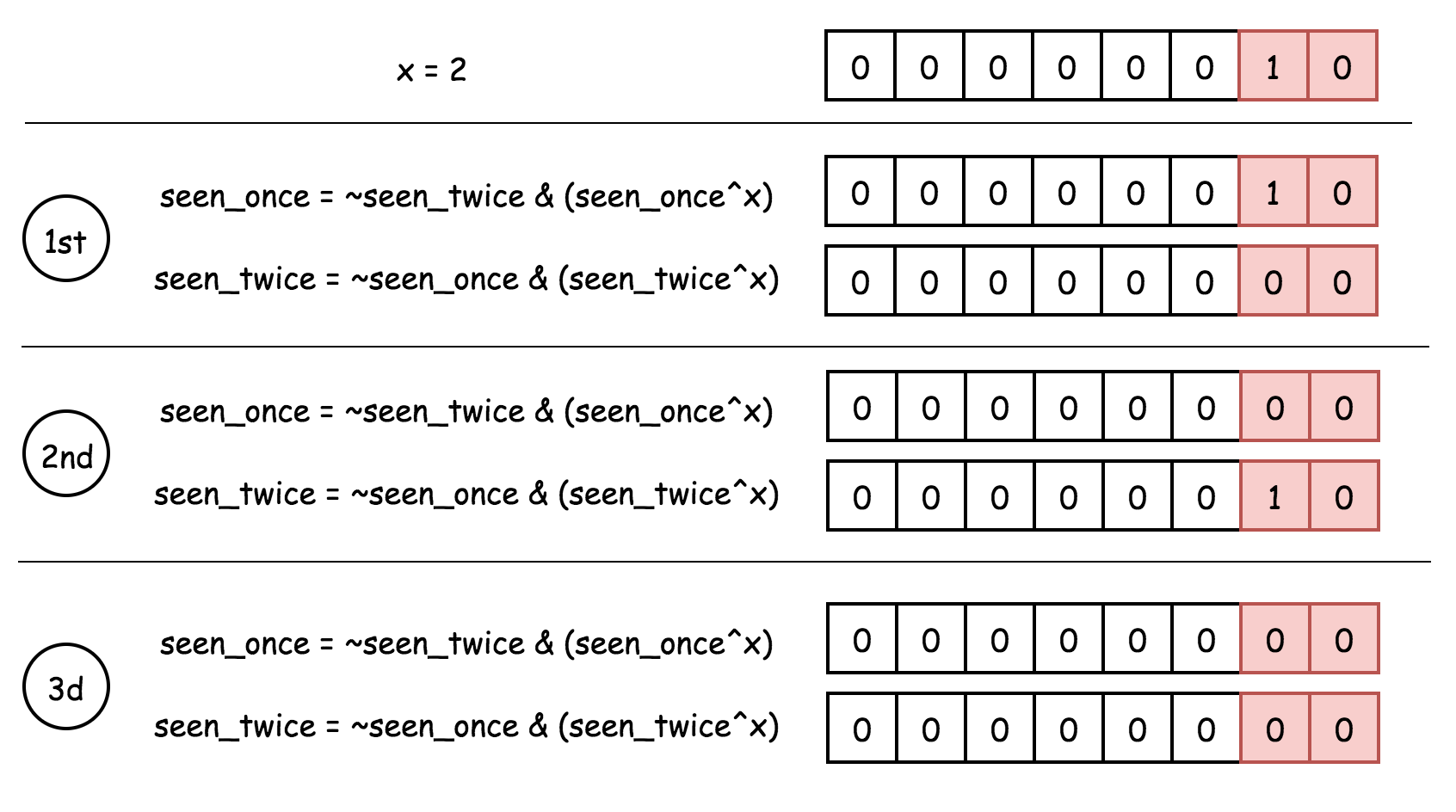# 剑指offer 56与57

## 0.137. 只出现一次的数字 II

method1：直接异或。

``````class Solution {
public:
int singleNumber(vector<int>& nums) {
int x = 0;
// 任何数与0异或是本身
// 任何数与本身亦或是0
// 现在有a,b,a两个数
// a^b=x
// x^a=b
// 根据这个性质我们得到如下实现
for(auto num : nums) {
x^=num;
}
return x;
}
};``````

## 1.面试题56 - I. 数组中数字出现的次数

``````class Solution {
public:
vector<int> singleNumbers(vector<int>& nums) {
int x=0;
// 遍历nums数组,得到异或结果
for(auto num : nums)
x^=num;
int h=1;
// 此时x必定是要求的两个数异或的结果，异或相同为0，不同为1，要区分开是哪一个数，我们只需要找出第一个位置是1的数就可以区分开。
while((x&h)==0)
h<<=1;
int a=0,b=0;
// 此时得到的这个数h必定与两个数中的某一个数&得0，可以区分出到底是那一个数，接下来分组就行。
for(auto num : nums)
if((h&num)==0)
a ^= num;
else
b ^= num;
return vector<int>{a,b};
}
};``````

## 2.面试题56 - II. 数组中数字出现的次数 II

method1：思路：转换成二进制，每一位的1加起来必定是3n或者3n+1，当是3n+1时候，这个1就是那个出现一次元素的1。3n的时候，把1消去，3n+1的时候记下来，就只剩下所求结果的二进制数，问题就转为二进制求十进制

``````class Solution {
public:
int singleNumber(vector<int>& nums) {
// 转换成二进制，每一位的1加起来必定是3n或者3n+1，当是3n+1时候，这个1就是那个出现一次元素的1
// 例如： 111
//       011 --> 所求结果
//       111
//       111
// 3n的时候，把1消去，3n+1的时候记下来，就只剩下所求结果的二进制数，问题就转为二进制求十进制
// 二进制求十进制方法:例如:11，就是2的1次方(2^1)异或0得到1,2^2异或1得到3，这便得到了结果。
int bitNum = {0}; // [高位...低位]
for(int i=0;i<nums.size();i++) {
int num = nums[i];
// 从后往前
for(int j=31;j>=0;j--) {
if((num&1) == 1) {
bitNum[j]++;
}
num >>= 1;
}
}
int res = 0;
int compare = 0;
// 3n/3n+1
for(int i=0;i<32;i++) {
res = res<<1;
res +=bitNum[i]%3;
}
return res;
}
};``````

method2：使用两个变量。``````class Solution {
public:
int singleNumber(vector<int>& nums) {
int one=0,two=0;
for(auto num:nums) {
one = ~two&(one^num);
two = ~one&(two^num);
}
return one;
}
};``````

## 3.面试题57. 和为s的两个数字

method1：双指针

``````class Solution {
public:
vector<int> twoSum(vector<int>& nums, int target) {
vector<int> res;
int l =0,r = nums.size()-1;
while(l<=r) {
if(nums[l]+nums[r] == target) {
res.push_back(nums[l]);
res.push_back(nums[r]);
break;
}
else if(nums[l]+nums[r]>target)
r--;
else
l++;
}
return res;
}
};``````

method2：不利用数组递增特性，使用set集合。

``````class Solution {
public:
vector<int> twoSum(vector<int>& nums, int target) {
set<int> s;
vector<int> res;
for(auto num : nums) {
if(s.count((target-num))>0) {
res.push_back(num);
res.push_back(target-num);
break;
}
s.insert(num);
}
return res;
}
};``````

## 4.面试题57 - II. 和为s的连续正数序列

``````class Solution {
public:
vector<vector<int>> findContinuousSequence(int target) {
vector<vector<int>> res;
int l=1,r=2;
while(l<r) {
int sum = (l+r)*(r-l+1)/2;
if (sum == target) {
vector<int> tmp;
for (int i=l;i<=r;i++) {
tmp.push_back(i);
}
res.push_back(tmp);
l++;
} else if(sum<target) {
r++;
} else {
l++;
}
}
return res;
}
};``````目录